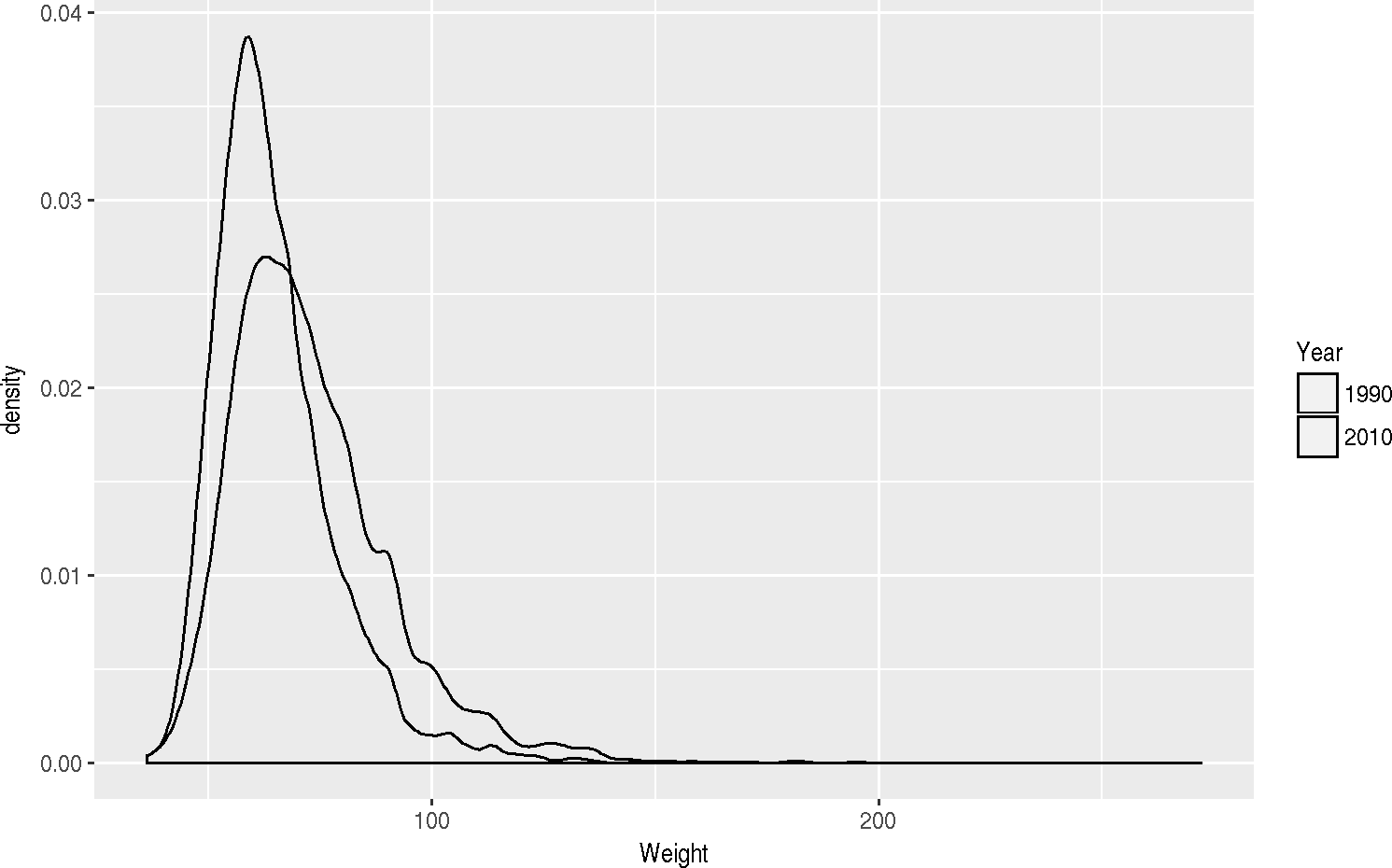# 1 Using R in real life

## 1.1 Organizing work

Usually, work is organized into a directory with:

• A folder containing R scripts (scripts/BRFSS-visualize.R)
• ‘External’ data like the csv files that we’ve been working with, usually in a separate folder (extdata/BRFSS-subset.csv)
• (sometimes) R objects written to disk using saveRDS() (.rds files) that represent final results or intermediate ‘checkpoints’ (extdata/ALL-cleaned.rds). Read the data into an R session using readRDS().
• Use setwd() to navigate to folder containing scripts/, extdata/ folder
• Source an entire script with source("scripts/BRFSS-visualization.R").

R can also save the state of the current session (prompt when choosing to quit() R), and to view and save the history() of the the current session; I do not find these to be helpful in my own work flows.

## 1.2R Packages

All the functionality we have been using comes from packages that are automatically loaded when R starts. Loaded packages are on the search() path.

search()
##   ".GlobalEnv"        "package:ggplot2"   "package:survival"
##   "package:BiocStyle" "package:stats"     "package:graphics"
##   "package:grDevices" "package:utils"     "package:datasets"
##  "package:methods"   "Autoloads"         "package:base"

Additional packages may be installed in R’s libraries. Use installed.packages() or the RStudio interface to see installed packages. To use these packages, it is necessary to attach them to the search path, e.g., for survival analysis

library("survival")

There are many thousands of R packages, and not all of them are installed in a single installation. Important repositories are

Packages can be discovered in various ways, including CRAN Task Views and the Bioconductor web and Bioconductor support sites.

To install a package, use install.packages() or, for Bioconductor packages, instructions on the package landing page, e.g., for GenomicRanges. Here we install the ggplot2 package.

install.packages("ggplot2", repos="https://cran.r-project.org")

A package needs to be installed once, and then can be used in any R session.

# 2 Graphics and Visualization

Load the BRFSS-subset.csv data

path <- "extdata/BRFSS-subset.csv"   # or file.choose()
brfss <- read.csv(path)

Clean it by coercing Year to factor

brfss$Year <- factor(brfss$Year)

## 2.1 Base R Graphics

Useful for quick exploration during a normal work flow.

• Main functions: plot(), hist(), boxplot(), …
• Graphical parameters – see ?par, but often provided as arguments to plot(), etc.
• Construct complicated plots by layering information, e.g., points, regression line, annotation.

brfss2010Male <- subset(brfss, (Year == 2010) & (Sex == "Male"))
fit <- lm(Weight ~ Height, brfss2010Male)

plot(Weight ~ Height, brfss2010Male, main="2010, Males")
abline(fit, lwd=2, col="blue")
points(180, 90, pch=20, cex=3, col="red")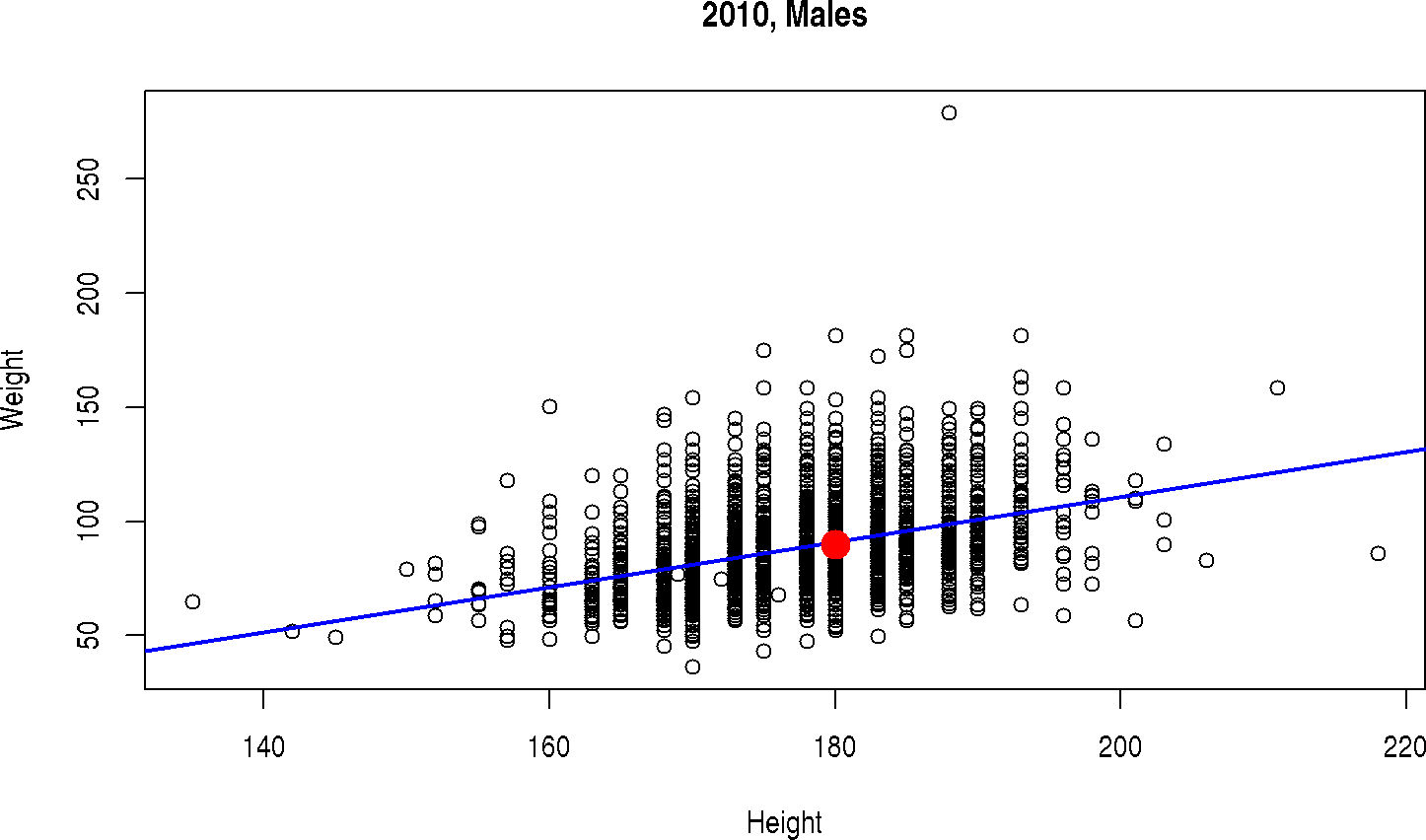• Approach to complicated graphics: create a grid of panels (e.g., par(mfrows=c(1, 2)), populate with plots, restore original layout.

brfssFemale <- subset(brfss, Sex=="Female")

opar = par(mfrow=c(2, 1))     # layout: 2 'rows' and 1 'column'
hist(                         # first panel -- 1990
brfssFemale[ brfssFemale$Year == 1990, "Weight" ], main = "Female, 1990") hist( # second panel -- 2010 brfssFemale[ brfssFemale$Year == 2010, "Weight" ],
main = "Female, 2010")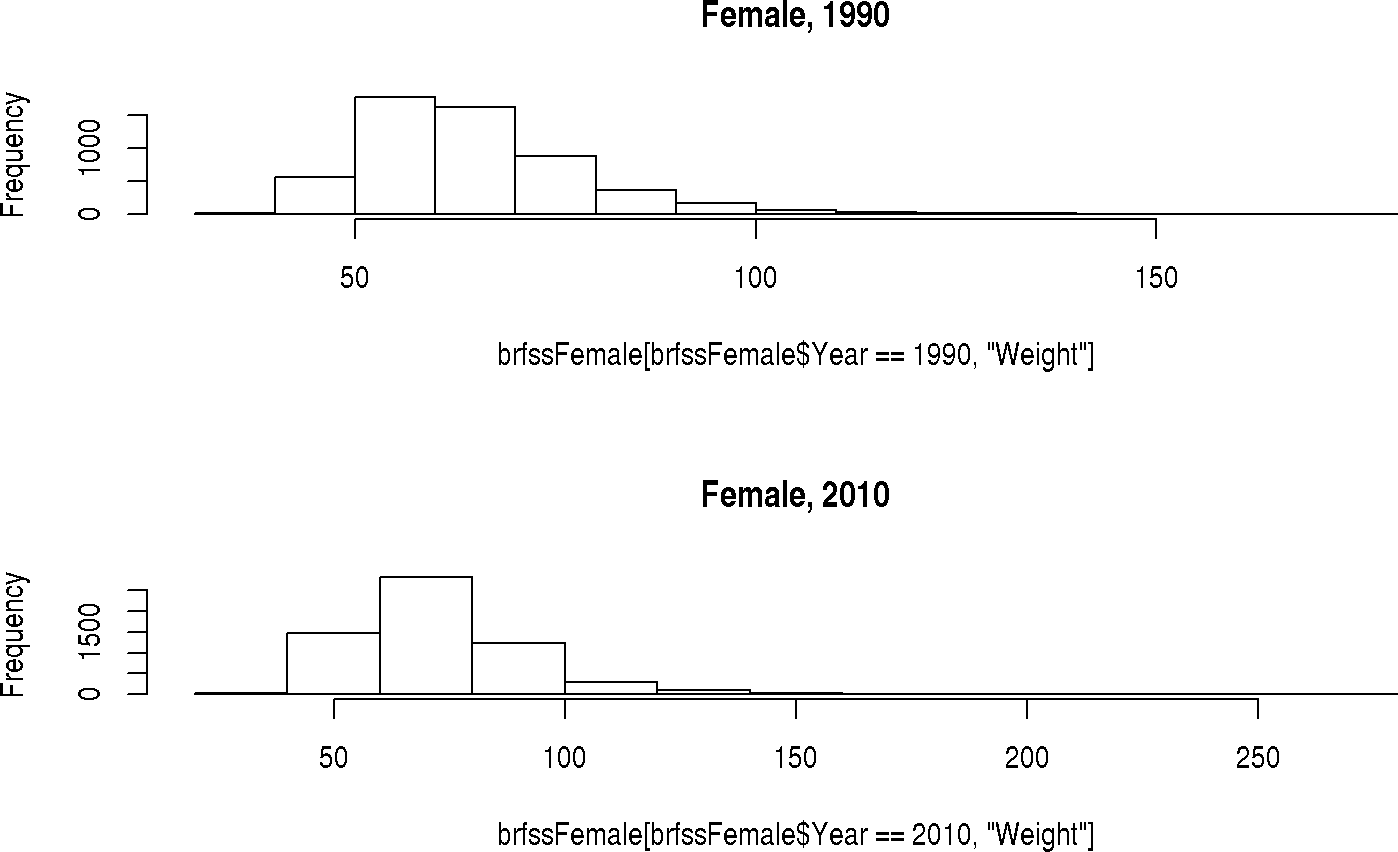par(opar)                      # restore original layout

## 2.2 What makes for a good graphical display?

• Common scales for comparison
• Efficient use of space
• Careful color choice – qualitative, gradient, divergent schemes; color blind aware; …
• Emphasis on data rather than labels
• Convey statistical uncertainty

## 2.3 Grammar of Graphics: ggplot2

library(ggplot2)

‘Grammar of graphics’

• Specify data and ‘aesthetics’ (aes()) to be plotted
• Add layers (geom_*()) of information

ggplot(brfss2010Male, aes(x=Height, y=Weight)) +
geom_point() +
geom_smooth(method="lm")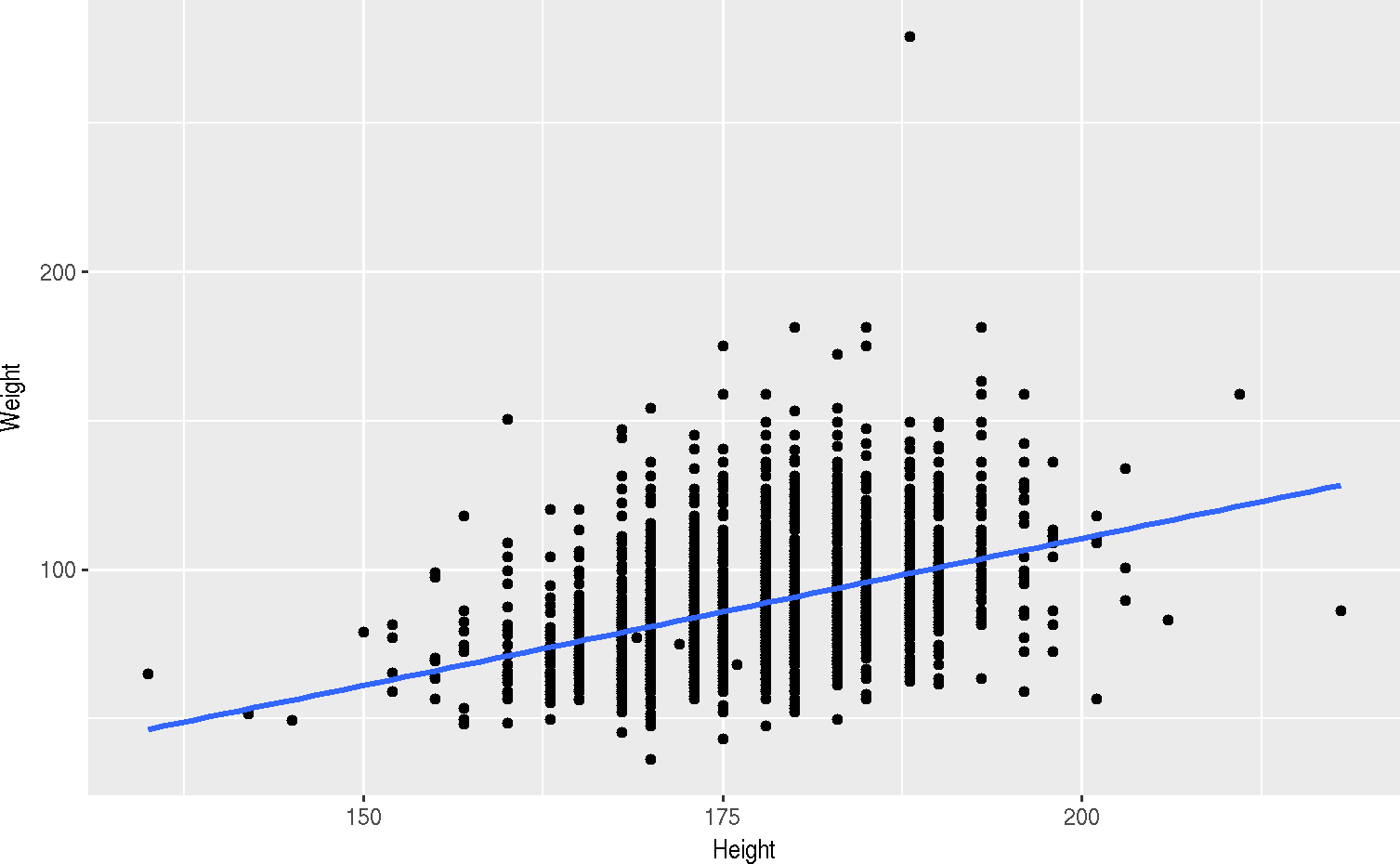• Capture a plot and augment it

plt <- ggplot(brfss2010Male, aes(x=Height, y=Weight)) +
geom_point() +
geom_smooth(method="lm")
plt + labs(title = "2010 Male")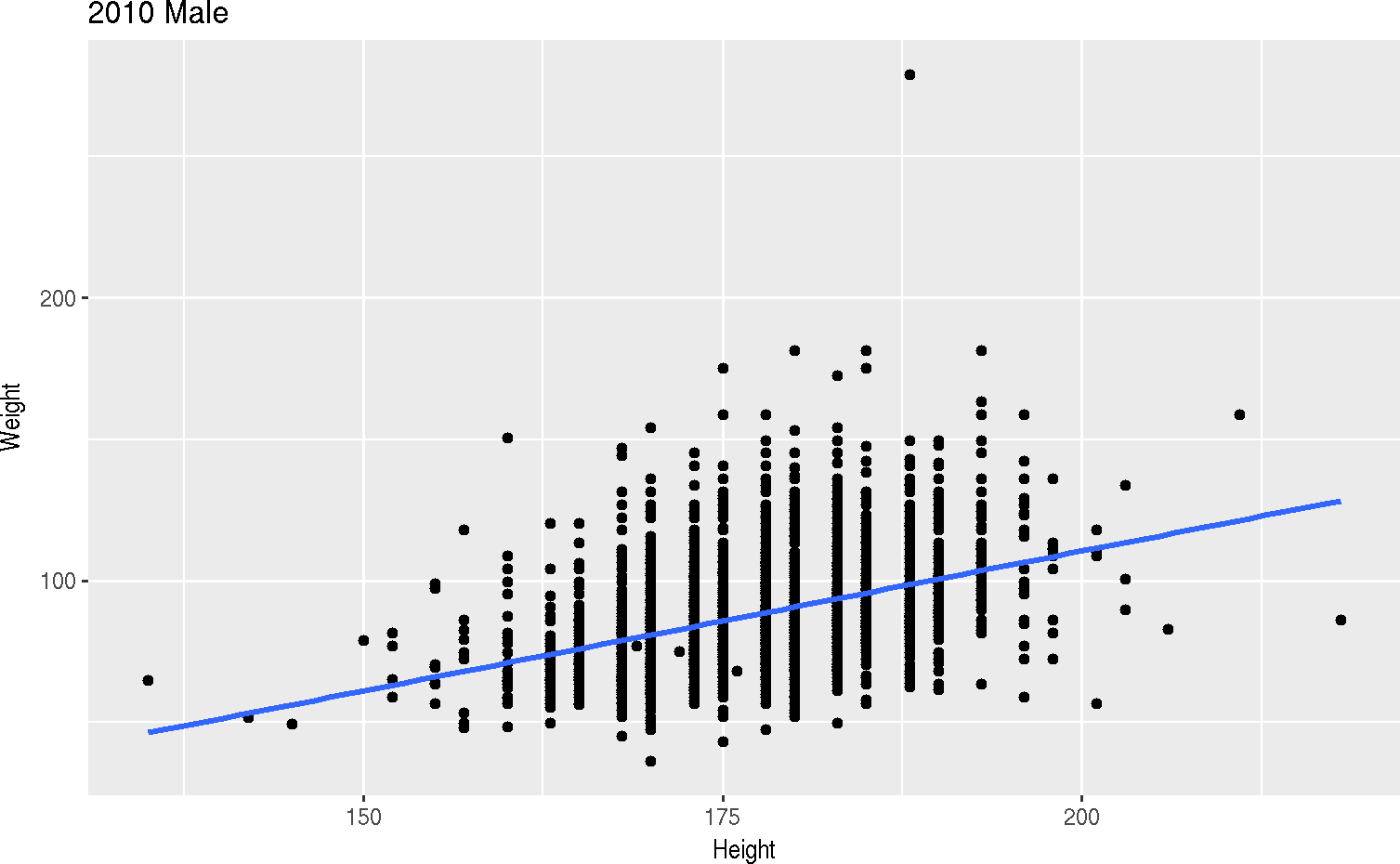• Use facet_*() for layouts

ggplot(brfssFemale, aes(x=Height, y=Weight)) +
geom_point() + geom_smooth(method="lm") +
facet_grid(. ~ Year)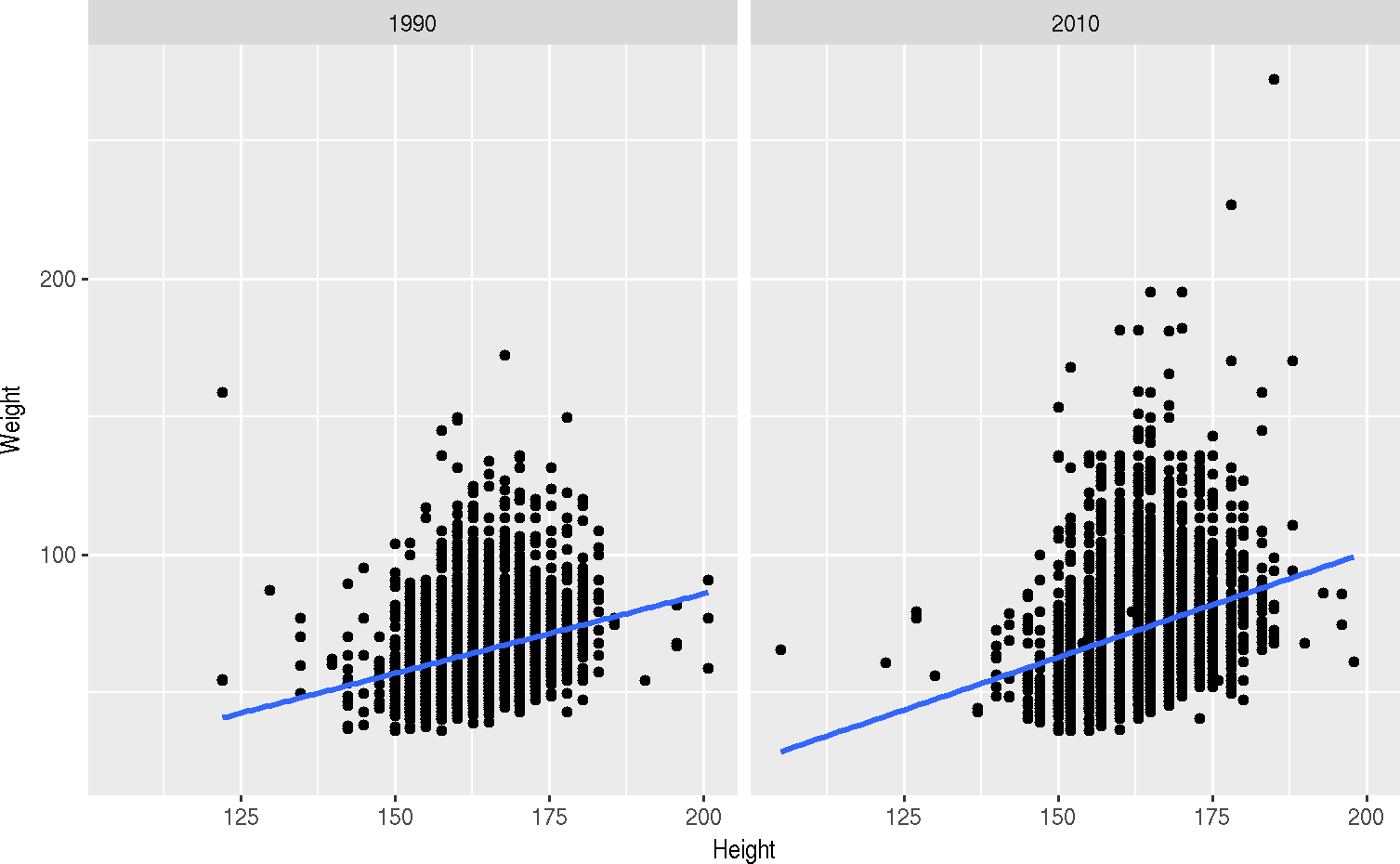• Choose display to emphasize relevant aspects of data

ggplot(brfssFemale, aes(Weight, fill=Year)) +
geom_density(alpha=.2)`